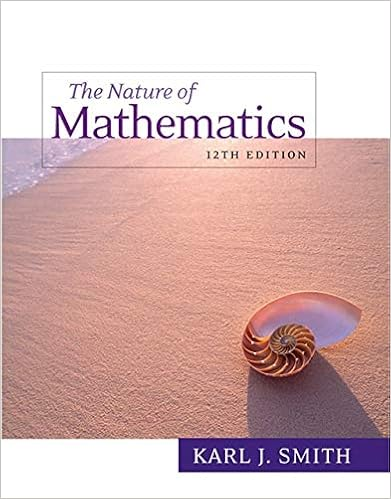# Is any value that separates the critical region where

• Notes
• 76
• 100% (4) 4 out of 4 people found this document helpful

This preview shows page 49 - 62 out of 76 pages.

##### We have textbook solutions for you!
The document you are viewing contains questions related to this textbook.The document you are viewing contains questions related to this textbook.
Chapter 17 / Exercise 10
Nature of Mathematics
SmithExpert Verified
is any value that separates the critical region (where we reject the null hypothesis) from the values of the test statistic that do not lead to rejection of the null hypothesis. The critical values depend on the nature of the null hypothesis, the sampling distribution that applies, and the significance level . See the previous figure where the critical value of z = 1.645 corresponds to a significance level of = 0.05.
##### We have textbook solutions for you!
The document you are viewing contains questions related to this textbook.The document you are viewing contains questions related to this textbook.
Chapter 17 / Exercise 10
Nature of Mathematics
SmithExpert Verified
Don’t confuse a P -value with a proportion p . Know this distinction: P -value = probability of getting a test statistic at least as extreme as the one representing sample data p = population proportion Types of Hypothesis Tests: Two-tailed, Left-tailed, Right-tailed P -value method : Using the significance level : If P -value , reject H 0 . If P -value > , fail to reject H 0 . Traditional method : If the test statistic falls within the critical region, reject H 0 . If the test statistic does not fall within the critical region, fail to reject H 0 .
Another option : Instead of using a significance level such as 0.05, simply identify the P -value and leave the decision to the reader. Confidence Intervals : A confidence interval estimate of a population parameter contains the likely values of that parameter. If a confidence interval does not include a claimed value of a population parameter, reject that claim. Wording of Final Conclusion Accept Versus Fail to Reject Some texts use “accept the null hypothesis.” We are not proving the null hypothesis. Fail to reject says more correctly The available evidence is not strong enough to warrant rejection of the null hypothesis (such as not enough evidence to convict a suspect).
Chapter 9 Inferences from Two Samples
Two samples are independent if the sample values selected from one population are not related to or somehow paired or matched with the sample values from the other population. Two samples are dependent if the sample values are paired . (That is, each pair of sample values consists of two measurements from the same subject (such as before/after data), or each pair of sample values consists of matched pairs (such as husband/wife data), where the matching is based on some inherent relationship.) Part 1: Independent Samples with σ 1 and σ 2 Unknown and Not Assumed Equal
Part 2: Alternative Methods Independent Samples with σ 1 and σ 2 Known. Methods for Inferences about Two Independent Means
Assume that σ 1 = σ 2 and Pool the Sample Variances. Requirements 1. The two population standard deviations are not known, but they are assumed to be equal. That is σ 1 = σ 2 . 2. The two samples are independent . 3. Both samples are simple random samples . 4. Either or both of these conditions are satisfied: The two sample sizes are both large (with n 1 > 30 and n 2 > 30) or both samples come from populations having normal distributions.
•••